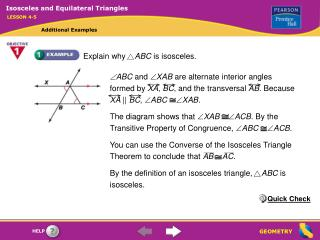DownloadDownload PresentationExplain why ABC is isosceles.

# Explain why ABC is isosceles.

Download Presentation## Explain why ABC is isosceles.

- - - - - - - - - - - - - - - - - - - - - - - - - - - E N D - - - - - - - - - - - - - - - - - - - - - - - - - - -
##### Presentation Transcript

1. ABC and XAB are alternate interior angles formed by XA, BC, and the transversal AB. Because XA || BC, ABCXAB. The diagram shows that XABACB. By the Transitive Property of Congruence, ABCACB. You can use the Converse of the Isosceles Triangle Theorem to conclude that ABAC. By the definition of an isosceles triangle, ABC is isosceles. Isosceles and Equilateral Triangles LESSON 4-5 Additional Examples Explain why ABC is isosceles. Quick Check

2. MOLNThe bisector of the vertex angle of an isosceles triangle is the perpendicular bisector of the base. x = 90 Definition of perpendicular Isosceles and Equilateral Triangles LESSON 4-5 Additional Examples Suppose that mL = y. Find the values of x and y. mN = mLIsosceles Triangle Theorem mL = y Given mN = yTransitive Property of Equality mN + mNMO + mMON = 180 Triangle Angle-Sum Theorem y + y + 90 = 180 Substitute. 2y + 90 = 180 Simplify. 2y = 90 Subtract 90 from each side. y = 45 Divide each side by 2. Quick Check Therefore, x = 90 and y = 45.

3. Isosceles and Equilateral Triangles LESSON 4-5 Additional Examples Suppose the raised garden bed is a regular hexagon. Suppose that a segment is drawn between the endpoints of the angle marked x. Find the angle measures of the triangle that is formed. Because the garden is a regular hexagon, the sides have equal length, so the triangle is isosceles. By the Isosceles Triangle Theorem, the unknown angles are congruent. Example 4 found that the measure of the angle marked x is 120°. The sum of the angle measures of a triangle is 180°. If you label each unknown angle y, 120 + y + y = 180. 120 + 2y = 180 2y = 60 y = 30 Quick Check So the angle measures in the triangle are 120°, 30° and 30°.